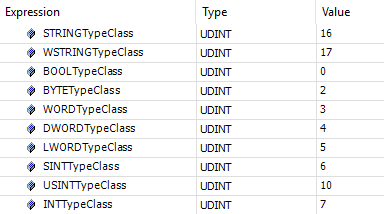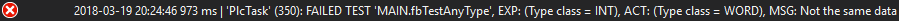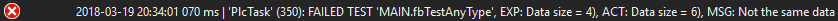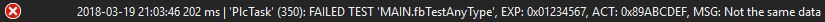While doing software development in TwinCAT, I have always been missing some sort of generic data type/container, to have some level of conformance to generic programming. “Generic programming… what’s that?”, you may ask. I like Ralf Hinze’s description of generic programming:

A generic program is one that the programmer writes once, but which works over many different data types.

I’ve been using generics in Ada and templates in C++, and many other languages have similar concepts. Why was there no such thing available in the world of TwinCAT/IEC 61131-3? For a long time there was a link to a type “ANY” in their data types section of TwinCAT 3, but the only information available on the website was that the “ANY” type was not yet available. By coincidence I revisited their web page to check it out, and now a description is available! I think the documentation has done a good job describing the possibilities with the ANY-type, but I wanted to elaborate with this a little further.

First of all, the documentation only mentions that the only current possibility to use the ANY-type as `VAR_INPUT` inside (free) functions, but I’ve found them to be working fine as `VAR_INPUT` in function block methods as well (though not as `VAR_INPUT` inside function block bodies). The example in the documentation is good, though I would like to make some adjustments and show a good use case for such an example.

The following example compares two ANY-types to check whether they are the same or not. This is useful in an unit testing framework, especially so in the case of IEC61131-3, as operator overloading is not available in the IEC standard. Assume we would like to write an assert function block, which does comparisons of two values and checks whether they are the same or not. As operator overloading is not available, this would require us to have different names depending on what data type we would like to compare, such as:

• `AssertEquals_BOOL(bExpected : BOOL; bActual : BOOL)`
• `AssertEquals_INT(nExpected : INT; nExpected : INT)`
• `AssertEquals_WORD(nExpected : WORD; nActual : WORD)`
• `AssertEquals_STRING(sExpected : STRING; sActual : STRING)`

Comparing this with most other programming languages where you can have operator overloading usually makes the code much cleaner. In a unit testing framework you might also want to add more, such as a message, but we’ll keep it simple for this example. If we have an assert function block with the above mentioned methods, we might complement this with:

• `AssertEquals(Expected : ANY; Actual : ANY)`

With this method we can provide any data type on the call of it, which makes it much more flexible. While it is simpler to do a simple comparison of the “basic” data types, normally by checking with the equality (=) or inequality (<>) operator for the data types, for the ANY types it requires us to do a little more work in the method body. But before trying to do any implementation, let’s look a little bit of how ANY works.

What happens in the background in TwinCAT is that when the code is compiled, TwinCAT internally replaces the any instances of ANY in functions/method with a structure which has the following contents:

``````TYPE AnyType :
STRUCT
// the type of the actual parameter
typeclass : __SYSTEM.TYPE_CLASS ;
// the pointer to the actual parameter
pvalue : POINTER TO BYTE;
// the size of the data, to which the pointer points
diSize : DINT;
END_STRUCT
END_TYPE
``````

That is really neat. Through this information, we can derive what data type and information lies within what the ANY-type is pointing to. The pointer basically points to the actual data that is defined as input for the method using the ANY-type as `VAR_INPUT`. But what is this `__SYSTEM.TYPE_CLASS`? If I do the classic “right-click” on the type, TwinCAT/Visual studio doesn’t give me any more hints or options to find out what it is. Let’s say I declare some variables with different types and send them into a method and store them into a variable holding the `__SYSTEM.TYPE_CLASS`. Doing this gives me different results for the variables, which is expected. I applied the following function on different types:

``````METHOD PRIVATE GetTypeClass : UDINT
VAR_INPUT
AnyData : ANY;
END_VAR

GetTypeClass := AnyData.TypeClass;
``````

And the result was as follows:Now the question arises – “Is every data type always represented with this number, independent of what compiler, target (x86, x64, ARM) or TwinCAT version that this runs on?”. What is more relevant to ask is – “Is there an enumeration that represents the different type classes?”. Thanks to the help of the local Beckhoff support I was pointed to the library “Base interfaces”, which indeed holds an enumeration for the different type classes:This is really great! With this information we can create a function that converts this enumeration into a string in case we for example want to utilize it for logging purposes.

``````FUNCTION F_AnyTypeClassToString : STRING
VAR_INPUT
AnyTypeClass : __System.TYPE_CLASS;
END_VAR

CASE UDINT_TO_INT(AnyTypeClass) OF
IBaseLibrary.TypeClass.TYPE_BOOL :
F_AnyTypeClassToString := 'BOOL';
IBaseLibrary.TypeClass.TYPE_BIT :
F_AnyTypeClassToString := 'BIT';
IBaseLibrary.TypeClass.TYPE_BYTE :
F_AnyTypeClassToString := 'BYTE';
IBaseLibrary.TypeClass.TYPE_WORD :
F_AnyTypeClassToString := 'WORD';
IBaseLibrary.TypeClass.TYPE_DWORD :
F_AnyTypeClassToString := 'DWORD';
IBaseLibrary.TypeClass.TYPE_LWORD :
F_AnyTypeClassToString := 'LWORD';
IBaseLibrary.TypeClass.TYPE_SINT :
F_AnyTypeClassToString := 'SINT';
IBaseLibrary.TypeClass.TYPE_INT :
F_AnyTypeClassToString := 'INT';
...
...
...
ELSE
F_AnyTypeClassToString := 'UNKNOWN';
END_CASE
``````

Now to get a feeling of how we could implement the `AssertEquals` method mentioned above, I’ll demonstrate how this piece of code could look like. First, the method header:

``````METHOD PUBLIC AssertEquals
VAR_INPUT
Expected : ANY;
Actual : ANY;
END_VAR
VAR
nCount : DINT;
bDataTypesNotEquals : BOOL := FALSE;
bDataSizeNotEquals : BOOL := FALSE;
bDataContentNotEquals : BOOL := FALSE;
sExpectedDataString : STRING(80);
sActualDataString : STRING(80);
END_VAR
``````

We declare three booleans to detect the three different use cases of when the two ANY data differs:

• When the types differ (e.g. real vs. int)
• When the size differ (e.g. 2 vs 4 bytes)
• When the content differ (e.g. 0x00A0 vs 0x00BD)

This can be realized with the following piece of code:

``````IF Expected.TypeClass <> Actual.TypeClass THEN
bDataTypesNotEquals := TRUE;
END_IF

IF NOT bDataTypesNotEquals THEN
IF (Expected.diSize <> Actual.diSize) THEN
bDataSizeNotEquals := TRUE;
END_IF
END_IF

IF NOT bDataTypesNotEquals AND NOT bDataSizeNotEquals THEN
// Compare each byte in the ANY-types
FOR nCount := 0 TO Expected.diSize-1 BY 1 DO
IF Expected.pValue[nCount] <> Actual.pValue[nCount] THEN
bDataContentNotEquals := TRUE;
EXIT;
END_IF
END_FOR
END_IF
``````

And to create an useful string that we can show a user, we need to write some additional code. What the below code basically does is that:

• First it checks if the types are not equal. If not, we print the two types. Otherwise we:
• Check if the size equals. If not, we print the two sizes. Otherwise we:
• Check if the content of the data is the same. If not, we print the (byte) content of the two ANY data
``````IF bDataTypesNotEquals THEN
sExpectedDataString := Tc2_Standard.CONCAT('(Type class = ', F_AnyTypeClassToString((Expected.TypeClass)));
sExpectedDataString := Tc2_Standard.CONCAT(sExpectedDataString, ')');
sActualDataString := Tc2_Standard.CONCAT('(Type class = ', F_AnyTypeClassToString(Actual.TypeClass));
sActualDataString := Tc2_Standard.CONCAT(sActualDataString, ')');
ELSIF bDataSizeNotEquals THEN
sExpectedDataString := Tc2_Standard.CONCAT('Data size = ', DINT_TO_STRING(Expected.diSize));
sExpectedDataString := Tc2_Standard.CONCAT(sExpectedDataString, ')');
sActualDataString := Tc2_Standard.CONCAT('Data size = ', DINT_TO_STRING(Actual.diSize));
sActualDataString := Tc2_Standard.CONCAT(sActualDataString, ')');
ELSIF bDataContentNotEquals THEN
FOR nCount := 0 TO MIN(Expected.diSize-1, 38) BY 1 DO // One byte will equal two characters (example: 255 = 0xff, 1 = 0x01)
sExpectedDataString := Tc2_Standard.CONCAT(STR1 := Tc2_Utilities.BYTE_TO_HEXSTR(in := Expected.pValue[nCount],
iPrecision := 2,
bLoCase := FALSE),
STR2 := sExpectedDataString);
END_FOR
sExpectedDataString := Tc2_Standard.CONCAT(STR1 := '0x', STR2 := sExpectedDataString);

FOR nCount := 0 TO MIN(Actual.diSize-1, 38) BY 1 DO // One byte will equal two characters (example: 255 = 0xff, 1 = 0x01)
sActualDataString := Tc2_Standard.CONCAT(STR1 := Tc2_Utilities.BYTE_TO_HEXSTR(in := Actual.pValue[nCount],
iPrecision := 2,
bLoCase := FALSE),
STR2 := sActualDataString);
END_FOR
sActualDataString := Tc2_Standard.CONCAT(STR1 := '0x', STR2 := sActualDataString);
END_IF

IF bDataTypesNotEquals OR bDataSizeNotEquals OR bDataContentNotEquals THEN
// Send the 'sActualDataString' to a logger...
END_IF
``````

Now I’ll demonstrate all three use cases with examples.

With example one I declare two different data types, `INT` and `WORD`, both with the same length (2 bytes).

``````VAR
ValueOne : INT := 15000;
ValueTwo : WORD := 120;
END_VAR
``````

Running the AssertEquals-code with the two variables above results in:How can we accomplish having two variables using the same data type but different sizes? Arrays! Remember, the ANY-type can literally take anything! We’ll declare:

``````VAR
ValueOne : ARRAY[1..2] OF INT;
ValueTwo : ARRAY[1..3] OF INT;
END_VAR
``````

And then we get:The first array consists of two integers (each 2 bytes, total of 4 bytes) while the other one consists of three integers (totalling 6 bytes), which is exactly what the message tells us.

``````VAR
Which gives the runresult: